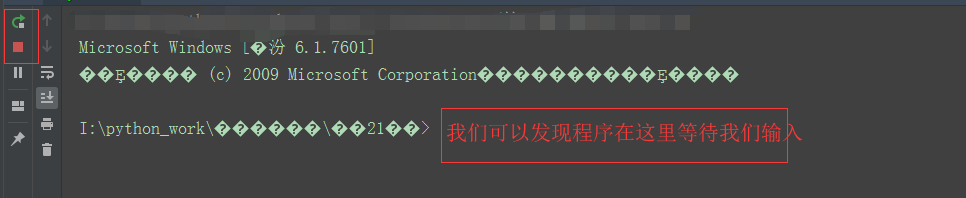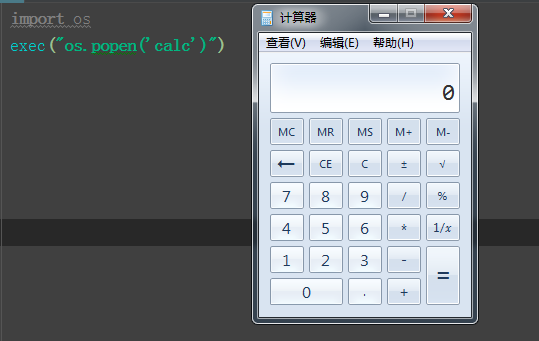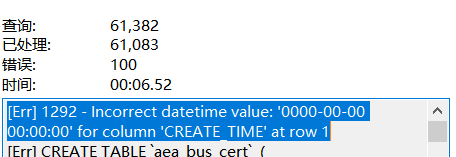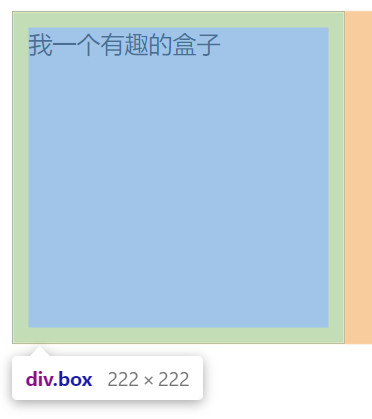Python装饰器、内置函数之金兰契友

• 对功能扩展开放：意味着有新的需求或变化时，可以对现有代码进行扩展，以适应新的情况。
• 对源码修改封闭：意味着类一旦设计完成，就可以独立完成其工作，而不要对源码进行任何修改。

``````def fun(function):  # 参数是接收装饰的函数
def decorator(*args,**kwargs):      # 装饰器
ret = function(*args,**kwargs)  # 接收最大数
print(f"最大的数是:{ret}")      # 将最大数打印出来
print(decorator)    # 查看装饰器与函数的区别
return decorator

# 假如num_max(num1,num2):是我们的源码，源码只是返回最大数。
# 现在有个需求让我们在不修改num_max(num1,num2)源码的情况下
# 以调用num_max(num1,num2)的方式将最大数打印出来
def num_max(num1,num2):
if num1 > num2:
return num1
else:
return num2
num_max = fun(num_max)  # 装饰函数num_max(num1,num2)
num_max(3,5)            # 以正常 的方式调用函数

# 打印结果：
<function fun.<locals>.decorator at 0x00000000021F47B8>

``````

Python为我们装饰函数的时候，提供了一个简便的方法语法糖@，可以直接装饰一个函数，还是以上面的例子演示：

``````def fun(function):  # 参数是接收装饰的函数
def decorator(*args,**kwargs):      # 装饰器
ret = function(*args,**kwargs)  # 接收最大数
print(f"最大的数是:{ret}")      # 将最大数打印出来
print(decorator)    # 查看装饰器与函数的区别
return decorator
# 假如num_max(num1,num2):是我们的源码，源码只是返回最大数。
# 现在有个需求让我们在不修改num_max(num1,num2)源码的情况下
# 以调用num_max(num1,num2)的方式将最大数打印出来
@fun    # 语法糖
def num_max(num1,num2):
if num1 > num2:
return num1
else:
return num2
num_max(3,5)   # 以正常 的方式调用函数

# 打印结果

``````

``````import time
def fun(function):  # 参数是接收装饰的函数
def decorator():      # 装饰器
start_time = time.time()
function()
end_time = time.time()
return (end_time - start_time)
return decorator

@fun    # 语法糖
def for_fun():
for i in range(10000000):
i += 1
ret = for_fun()
print(f"for循环用了：{ret}")

@fun    # 语法糖
def while_fun():
count = 0
while count < 10000000:
count += 1
ret = while_fun()
print(f"while循环用了：{ret}")

# 打印内容如下
for循环用了：0.7150406837463379
while循环用了：0.7400426864624023
``````

``````from functools import wraps

def inner(func):
@wraps(func) #加在最内层函数正上方
def wrapper(*args,**kwargs):
return func(*args,**kwargs)
return wrapper

@inner
def func():
'''我是注释'''
print('from index')

print(func.__doc__)
print(func.__name__)
``````

abs(x)：x是一个数，函数返回一个绝对值。

``````print(abs(-9))
# 打印内容如下:
9
``````

all(iterable)：参数,可迭代对象，检查可迭代对象中的元素是否为True，如果元素中有False、None、0、""空字符串返回False，否则返回True。

``````print(all([1,2,"a"]))
print(all([1,"a",2,""]))
print(all([1,"a",2,None]))
print(all([1,"a",2,0]))

# 打印内容如下:
True
False
False
False
``````

any(iterable)：参数是可迭代对象，如果可迭代对象是空返回False，否则返回True。

``````print(any([1,2,"",False]))
print(any([]))

# 打印内容如下:
True
False
``````

bin(x)：参数是数字，将数字转换成二进制。

``````print(bin(4))

# 打印内容如下:
0b100
``````

chr(i)：参数i是一个数字，范围是0 - 1,114,111转换成Unicode码

``````print(chr(97))

# 打印内容如下:
a
``````

oct(x)：x是一个数字,返回这个数字的八进制。
hex(x)：x是一个数字,返回这个数字的十六进制。
ord(c)：返回一个字符的Unicode对应的数字。
delattr(object, name)：参数object是一个对象，name是对象的属性，功能删除对象的属性。

``````class A:
def __init__(self,name):
self.name = name
obj = A("小明")
print(obj.__dict__) # 打印对象所有属性
delattr(obj, 'name') # 删除对象属性
print(obj.__dict__) # 打印对象所有属性
# 打印内容如下:
{'name': '小明'}
{}
``````

divmod(a,b)：参数a是一个数字，参数b是一个数字，计算a除b返回(商和余数)。

``````print(divmod(11,3))

#打印内容如下:
(3, 2)
``````

eval(expression, globals=None, locals=None)：实际项目中最好不要用，因为它可以将表达式的参数解析成Python语句执行，这样用户就可以通过eveal()来对我们的程序进行一些不可预知的操作。
expression：表达式
globals -- 变量作用域，全局命名空间，如果被提供，则必须是一个字典对象。
locals -- 变量作用域，局部命名空间，如果被提供，可以是任何映射对象。

``````import os
eval("os.system('cmd.exe')")
````````````# 假设这是我们源码中的函数
def fun():
print("我是fun")

# 用户无意间输入了"fun()"并传给了eval
eval("fun()")

# 打印结果如下

``````

``````dict_1 = {"a":1,"b":2}
str_1 = str(eval("dict_1")) # 将字典转换成字符串
dict_2 = dict(eval(str_1)) # 将字符串转换成字典
print(str_1,type(str_1))
print(dict_2,type(dict_2))

# 打印内容如下:
{'a': 1, 'b': 2} <class 'str'>
{'a': 1, 'b': 2} <class 'dict'>
``````

exec(object,globals,locals)：这个也是个神奇的家伙，与eval类似可以动态执行Python代码，实际开发中也是禁止使用的。

``````import os
exec("os.popen('calc')")  # 打开系统自带的计算器
````````````exec("for i in range(5):print(i,end=\" \")")

# 打印内容
0 1 2 3 4
``````

help(object)：返回对象的帮助页面。

``````help(print)

# 打印内容如下:
print(...)
print(value, ..., sep=' ', end='\n', file=sys.stdout, flush=False)

Prints the values to a stream, or to sys.stdout by default.
Optional keyword arguments:
file: a file-like object (stream); defaults to the current sys.stdout.
sep: string inserted between values, default a space.
end: string appended after the last value, default a newline.
flush: whether to forcibly flush the stream.

print(self, *args, sep=' ', end='\n', file=None)
``````

print(self, *args, sep=' ', end='\n', file=None)：

*args：用户传入的参数。
sep：当用户输入多个用逗号","分隔的参数时，默认sep是空格分开每个值,可以指定sep="_"等其它符号来对值进行分隔。例如：

``````print("Hello","World","!!!",sep="_|_")

# 打印内容如下:
Hello_|_World_|_!!!
``````

end：用于指定每个print结尾，默认是换行符，也就是每个print占一行可以指定参数让print不进行换行，如下：

``````print("Hello","World","!!!",sep="_|_",end="****")
print("无语呀")

# 打印内容如下:
Hello_|_World_|_!!!****无语呀
``````

hash(对象)：获取对象的hash值。
id(对象)：获取对象内存地址。
round(*args, **kwargs)： *args：是一个小数  **kwargs：用于控制小数的位数，如果不填写默认只显示整数。

``````print(round(1.2345))
print(round(1.2345,2))

# 打印结果如下
1
1.23
``````

pow(a,b) ：求a的b次幂，如果有个三次参数，则求完次幂后对第三方个数取余。
sum() ：求和。
max() ：求最大值。
enumerate() ：获取枚举对象，并列出下标。

``````list_ = [1,2,3]
for i,k in enumerate(list_):
print(i,k,end=" ")

print("\n")

for i in enumerate(list_):
print(i,end=" ")

# 打印结果如下
0 1 1 2 2 3

(0, 1) (1, 2) (2, 3)
``````

dir(object) ：如果没有参数，则返回当前局部作用域中的名称列表。使用参数，尝试返回该对象的有效属性列表。

``````list_ = [1,2,3]
print(dir(list_))
print(dir(input))

# 打印内容如下:
['__add__', '__class__', '__contains__', '__delattr__', '__delitem__', '__dir__', '__doc__', '__eq__', '__format__', '__ge__', '__getattribute__', '__getitem__', '__gt__', '__hash__', '__iadd__', '__imul__', '__init__', '__init_subclass__', '__iter__', '__le__', '__len__', '__lt__', '__mul__', '__ne__', '__new__', '__reduce__', '__reduce_ex__', '__repr__', '__reversed__', '__rmul__', '__setattr__', '__setitem__', '__sizeof__', '__str__', '__subclasshook__', 'append', 'clear', 'copy', 'count', 'extend', 'index', 'insert', 'pop', 'remove', 'reverse', 'sort']
['__call__', '__class__', '__delattr__', '__dir__', '__doc__', '__eq__', '__format__', '__ge__', '__getattribute__', '__gt__', '__hash__', '__init__', '__init_subclass__', '__le__', '__lt__', '__module__', '__name__', '__ne__', '__new__', '__qualname__', '__reduce__', '__reduce_ex__', '__repr__', '__self__', '__setattr__', '__sizeof__', '__str__', '__subclasshook__', '__text_signature__']
``````

callable() ：用于检查一个对象是否是可调用的，如果返回True，object有可能调用失败，要是返回False调用失败。

``````def num_sum(a,b):
return a+b
print(num_sum(1,2))
print(callable(num_sum))

# 打印内容如下:
3
True
``````

zip()： 函数用于将可迭代的对象作为参数，将多个对象中对应的元素打包成一个元祖，然后返回由这些元祖组成的内容，如果各个迭代器的元素个数不一致，则按照长度最短的返回。

``````list_ = [1,2,3]
list_1 = ["a","b","c"]
list_2 = ["1","2","3"]
print(list(zip(list_,list_1,list_2)))

# 打印内容如下:
[(1, 'a', '1'), (2, 'b', '2'), (3, 'c', '3')]
``````

lambda匿名函数：

1、函数的参数可以有多个，多个参数之间用逗号隔开。
2、匿名函数不管多复杂，只能写一行，且逻辑结束后直接返回数据。
3、返回值和正常的函数一样，可以是任意数据类型，但是只能一个，不能返回多个。

``````# 示例1合并列表
x=(1,3,5)
y=(0,2,4)

list_1 = list(map(lambda x,y:(x,y),y,x))
print(list_1)

# 打印内ring如下：
[(0, 1), (2, 3), (4, 5)]

# 示例2 对列表进行排序
list_1 = [(1,2),(3,5),(8,4),(6,1)]
print(sorted(list_1,key=lambda x:x))
print(list_1)

# 打印内容如下
[(6, 1), (1, 2), (8, 4), (3, 5)]
[(1, 2), (3, 5), (8, 4), (6, 1)]
``````

sorted排序函数

iterable：可迭代对象。
key：排序规则(函数)，在sorted内部会将可迭代对象中的每一个元素传递给这个函数作为参数，根据函数运算的结果进行排序。
reverse：是否是倒序，True 倒叙 False 正序。

``````# 示例1
array = [{"age":20,"name":"a"},{"age":25,"name":"b"},{"age":10,"name":"c"}]
print(sorted(array,key=lambda k:k["age"]))  # 默认是升序排序

# 打印内容如下:
[{'age': 10, 'name': 'c'}, {'age': 20, 'name': 'a'}, {'age': 25, 'name': 'b'}]

# 示例2
array = [{"age":20,"name":"a"},{"age":25,"name":"b"},{"age":10,"name":"c"}]
print(sorted(array,key=lambda k:k["age"]*-1))  # 在值后面*-1和reverse=True类似倒叙排列

# 打印内容如下:
[{'age': 25, 'name': 'b'}, {'age': 20, 'name': 'a'}, {'age': 10, 'name': 'c'}]

# 示例3
list_1 = [(1,2),(3,5),(8,4),(6,1)]
print(sorted(list_1,key=lambda x:x,reverse=True))
print(list_1)

# 打印内容如下
[(3, 5), (8, 4), (1, 2), (6, 1)]
[(1, 2), (3, 5), (8, 4), (6, 1)]
``````

function：用来筛选的函数，在filter中会自动的把iterable中的元素传递给function，然后根据function返回的True或者False来判断是否保留此项数据。
iterable：可迭代对象。

``````array = [{"age":20,"name":"a"},{"age":25,"name":"b"},{"age":10,"name":"c"}]
# 过滤掉年龄小于10的
print(list(filter(lambda k:k["age"]>10,array)))

# 打印内容如下:
[{'age': 20, 'name': 'a'}, {'age': 25, 'name': 'b'}]
``````

``````list_1 = [1,2,3,4,5]
print(list(map(lambda x:x**2,list_1)))

# 打印内容如下
[1, 4, 9, 16, 25]
``````

``````# 示例1
lst = [10,2,30,4,5,6,70,8,9]
print(list(map(lambda x:x>10,lst))) # 如果是条件表达式则返回的是
# True或者是Fales

# 打印内容如下:
[False, False, True, False, False, False, True, False, False]

# 示例2
lst1 = list(range(0,6,2))
lst2 = list(range(1,6,2))
print(list(map(lambda x,y:(x,y),lst1,lst2)))

# 打印内容如下:
[(0, 1), (2, 3), (4, 5)]
``````

reduce(function, iterable[, initializer) 函数会对参数序列中元素进行累积。

function：函数，有两个参数
iterable ：可迭代对象
initializer ： 可选，初始参数

``````# 示例1
from functools import reduce
list_1 = [1,2,3,4,5]
print(reduce(lambda x,y:x+y,list_1))

# 打印内容如下
15

# 示例2
str_1 = "abcabbcacda"
word_count =reduce(lambda a,x:a+x.count("a"),str_1,0)
print(word_count)

# 打印内容如下
4
``````浅梦一笑
5个月前blmius
1年前
MySQL:[Err] 1292 - Incorrect datetime value: ‘0000-00-00 00:00:00‘ for column ‘CREATE_TIME‘ at row 1刚刚好
5个月前
css问题晴空闲云
5个月前
css中box-sizing解放盒子实际宽高计算Easter79
1年前
swap空间的增减方法
（1）增大swap空间去激活swap交换区：swapoff v /dev/vg00/lvswap扩展交换lv：lvextend L 10G /dev/vg00/lvswap重新生成swap交换区：mkswap /dev/vg00/lvswap激活新生成的交换区：swapon v /dev/vg00/lvswapStella981
1年前
Python之time模块的时间戳、时间字符串格式化与转换
Python处理时间和时间戳的内置模块就有time，和datetime两个，本文先说time模块。关于时间戳的几个概念时间戳，根据1970年1月1日00:00:00开始按秒计算的偏移量。时间元组（struct_time），包含9个元素。 time.struct_time(tm_yWesley13
1年前
Java日期时间API系列36
十二时辰，古代劳动人民把一昼夜划分成十二个时段，每一个时段叫一个时辰。二十四小时和十二时辰对照表：时辰时间24时制子时深夜11：00凌晨01：0023：0001：00丑时上午01：00上午03：0001：0003：00寅时上午03：00上午0Wesley13
1年前
PHP中的NOW（）函数Wesley13
1年前
MySQL部分从库上面因为大量的临时表tmp_table造成慢查询helloworld_34035044
8个月前

​在我们用皕杰报表工具设计填报报表时，如何在新增行里自动增加id呢？能新增整数排序id吗？目前可以在新增行里自动增加id，但只能用uuid函数增加UUID编码，不能新增整数排序id。uuid函数说明：获取一个UUID，可以在填报表中用来创建数据ID语法：uuid()或uuid(sep)参数说明：sep布尔值，生成的uuid中是否包含分隔符''，缺省为Stella981
Lv1

1.7w

29

9